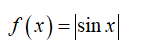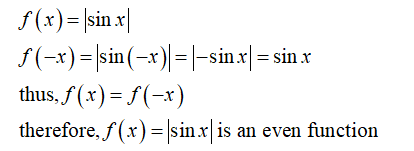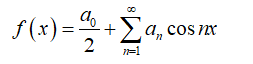# Sketch the function f(x) = |sin(x)| and find a Fourier series representation of it.

Question
1 views

Sketch the function f(x) = |sin(x)| and find a Fourier series representation of it.

check_circle

Step 1

According to the given information it is required to find the Fourier series of the function:Step 2

First, find whether the given function is odd or even. If a function is odd then, f(-x) = -f(x) and if a function is even then f(-x) = f(x).

So, find whether the given function if odd or even.Step 3

The Fourier series of an even function contains only cosine terms, and the Fourier series is given by:...

### Want to see the full answer?

See Solution

#### Want to see this answer and more?

Solutions are written by subject experts who are available 24/7. Questions are typically answered within 1 hour.*

See Solution
*Response times may vary by subject and question.
Tagged in

### Math